# Dynamics on q-vol lozenge tilings inverting the parameter q

### The model 

We consider the measure on lozenge tilings of a hexagon in which the probabilistic weight of every tiling is proportional to $q^{volume}$ or $q^{-volume}$, where $0< q <1$. The dynamics starts from an exact sample of the measure $q^{-volume}$ (which is produced by Vadim Gorin’s program ). Then, by randomly moving vetical lozenges to the left, the measure $q^{-volume}$ becomes the measure $q^{volume}$.

### Sampling algorithm

The simulation is based on a bijectivisation of the Yang-Baxter equation . Its details are explained in the forthcoming publication .

### Pictorial results

Here is a sample of three random tilings of the hexagon of size $50\times 50\times 50$ in the beginning, midway, and in the end of the simulation. Throughout the simulation, each random configuration has a distribution which is of $q$-Gibbs type, but with powers of $q$ reshuffled (these are the parameters in the corresponding skew Schur factors).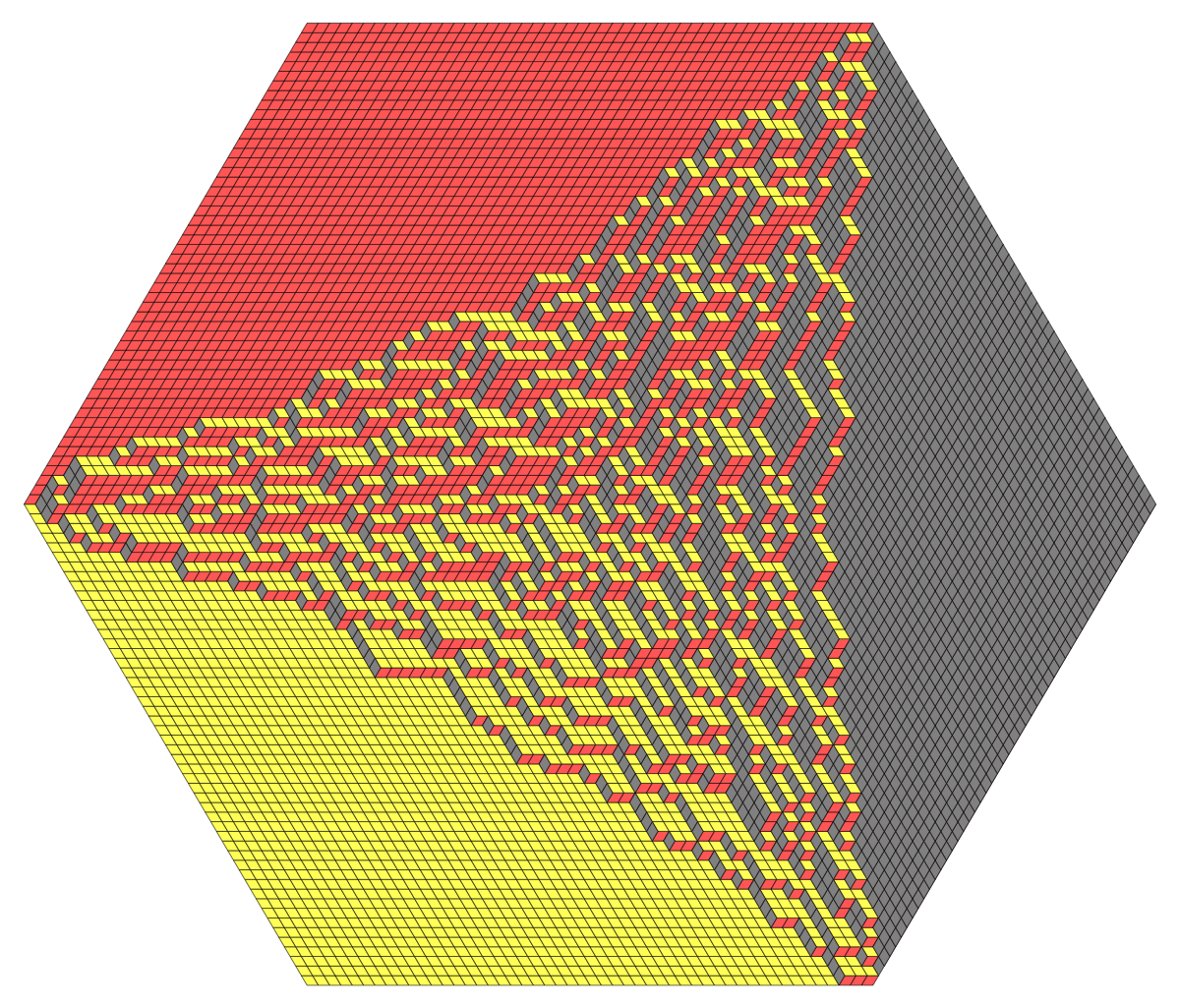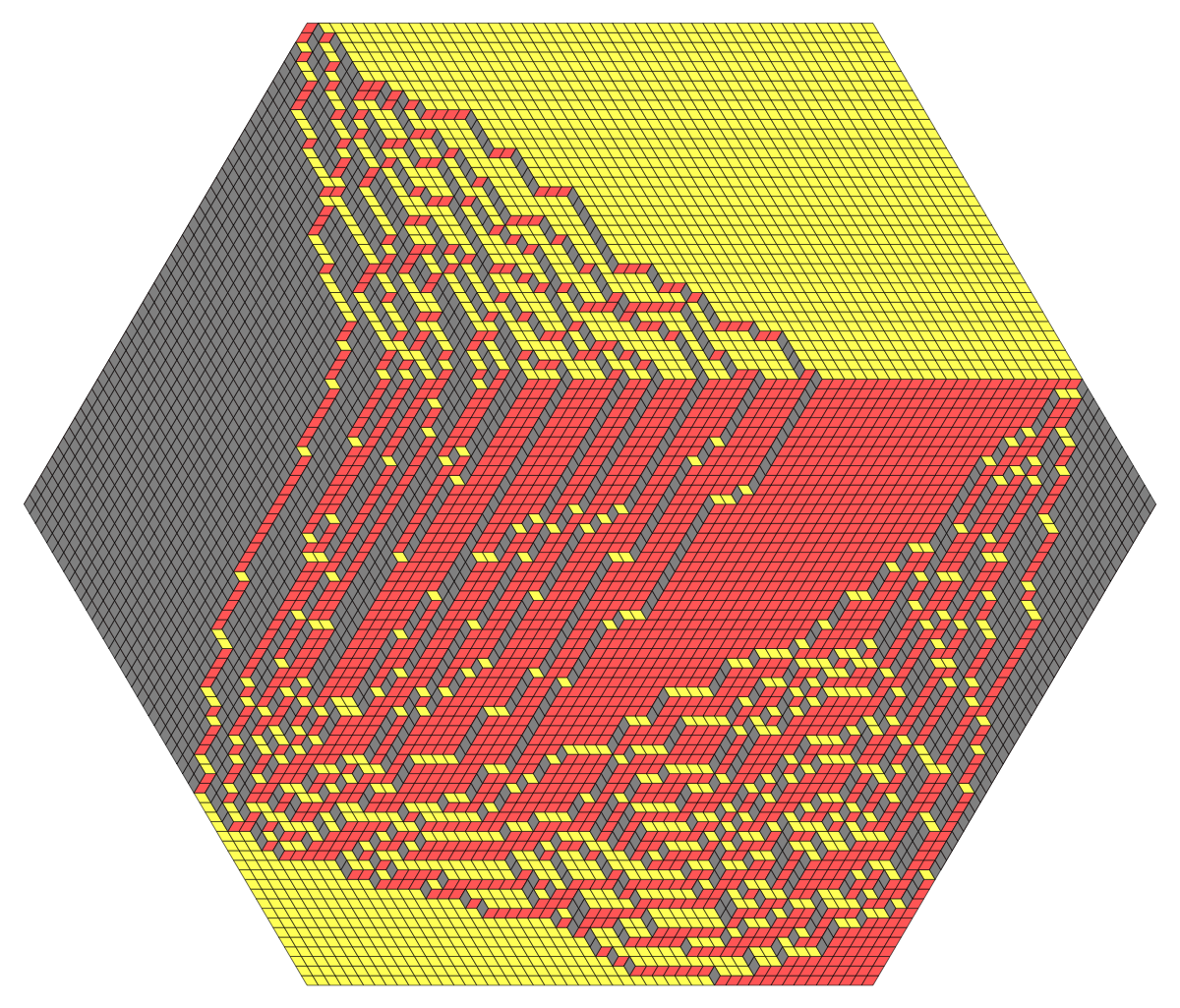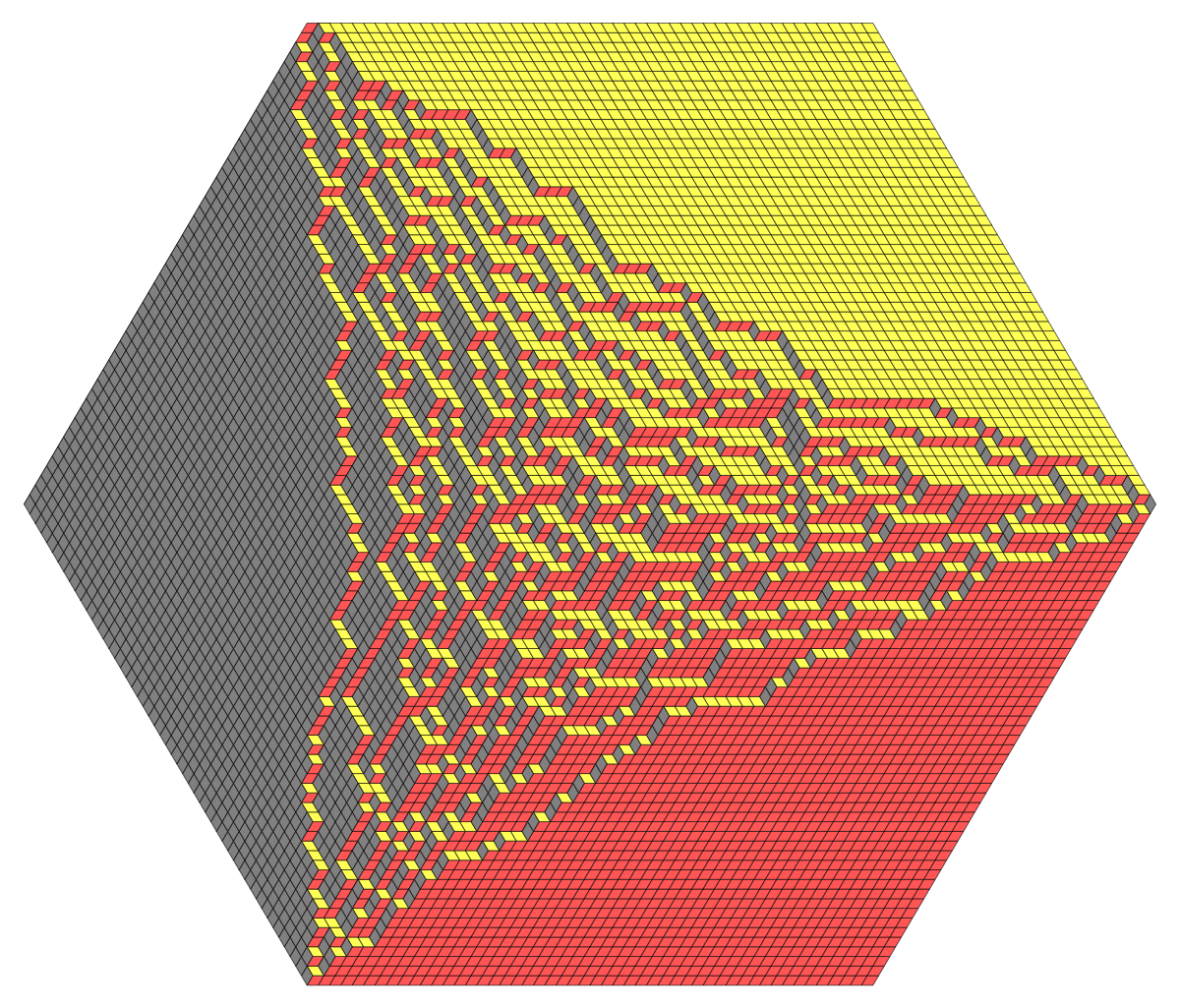### Data file format

The data file is a list of lists of lists in Mathematica-readable format, of the form $\{ \lambda(1),\lambda(2),\ldots,\lambda(T) \},$ where each $\lambda(t)$ is a list of weakly interlacing integer coordinates of the form $\{ \{ 47 \},\{ 50,47 \} , \{ 50,49,47 \} ,\ldots, \} .$ Here $t$ is the time variable. The simulation data can be “coarse” in larger tilings, or finer with every step of the Markov chain recorded.

The simulations are gifs or movies.

## code • (Main GitHub repo)

(note: parameters in the code might differ from the ones in simulation results below)

## simulation results

1. ##### A gif example of a dynamics of only vertical lozenges • (graphics: 18 MB)
Hexagon $10\times 10\times 10$, $q=0.85$2. ##### GIF of a dynamics of only vertical lozenges • (graphics: 18 MB)
Hexagon $10\times 10\times 10$, $q=0.85$3. ##### Movie, size 10, all frames • (graphics: 300 KB)
Hexagon $10\times 10\times 10$, $q=0.85$.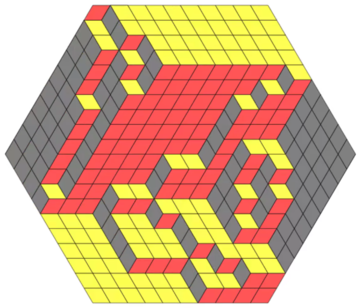4. ##### Movie, size 25, all frames. Almost frozen configuration • (graphics: 1.2 MB)
Hexagon $25\times 25\times 25$, $q=0.7$.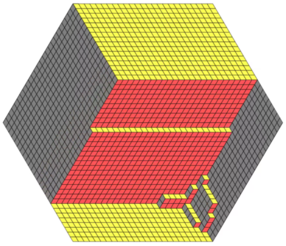5. ##### Movie, size 50, only frames after each sweep • (data: 1.3 MB) • (graphics: 2.4 MB)
Hexagon $50\times 50\times 50$, $q=0.95$.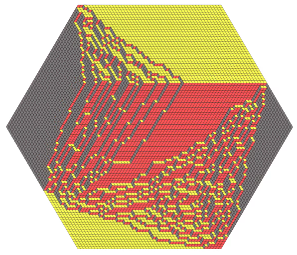6. ##### Movie, size 50, all frames • (data: 63.6 MB) • (graphics: 7.3 MB)
Hexagon $50\times 50\times 50$, $q=0.95$. Took several days to render all the 5K frames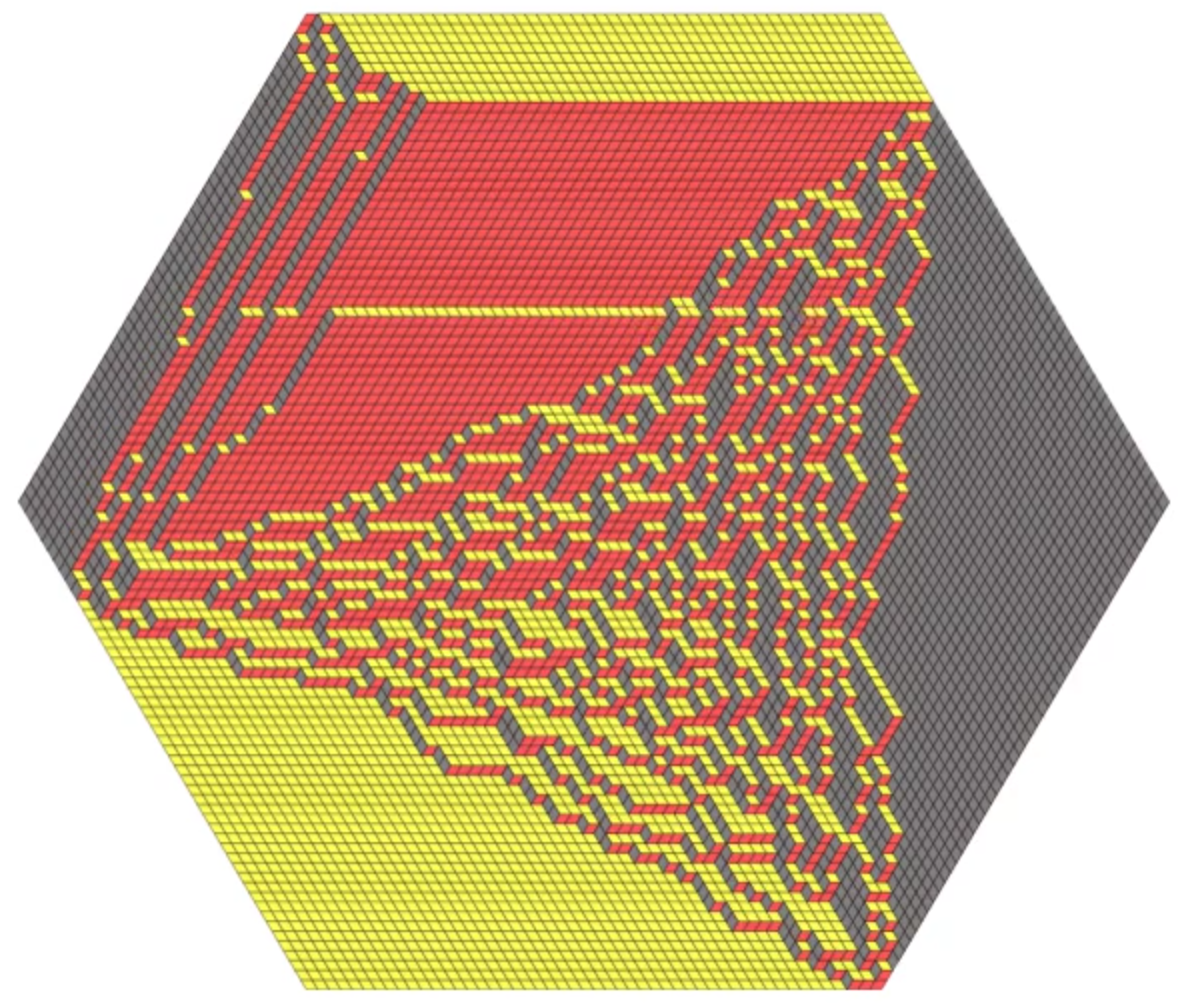7. ##### Movie, size 100, only frames after each sweep • (data: 11.2 MB) • (graphics: 24.1 MB)
Hexagon $100\times 100\times 100$, $q=0.95$.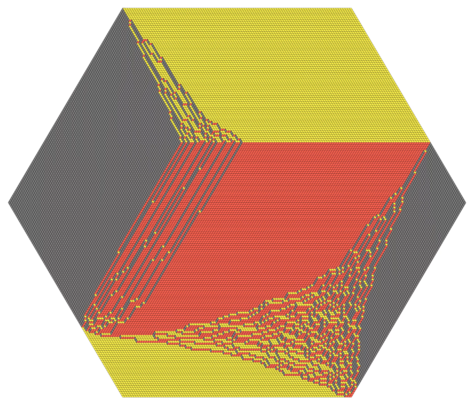## references

1. L. Petrov, A. Saenz. In preparation (2019)
2. A.Borodin, V. Gorin. Shuffling algorithm for boxed plane partitions. Advances in Mathematics, 220 (6) (2009). 1739-1770, • https://arxiv.org/abs/0804.3071https://www.sciencedirect.com/science/article/pii/S0001870808003253
3. A. Bufetov, L. Petrov. Yang-Baxter field for spin Hall-Littlewood symmetric functions (2017) • https://arxiv.org/abs/1712.04584